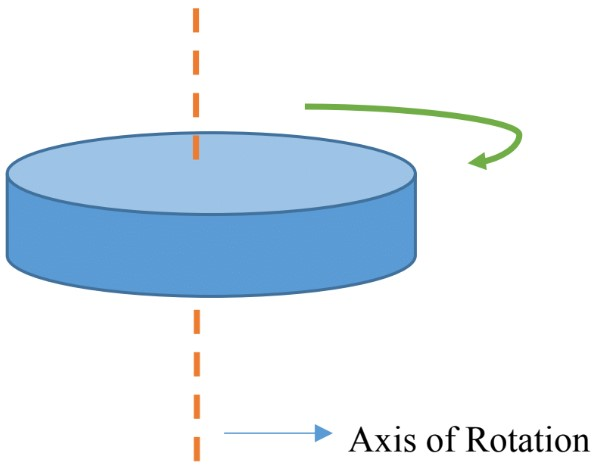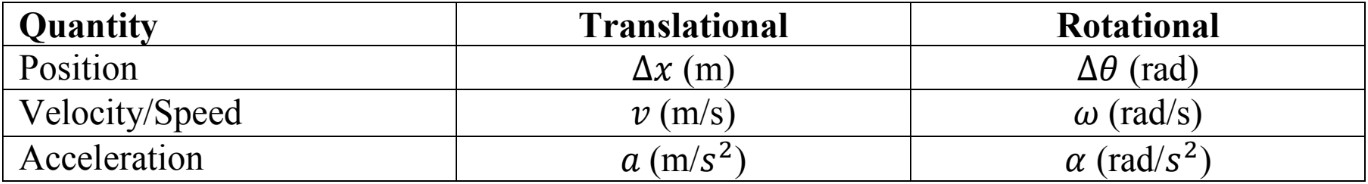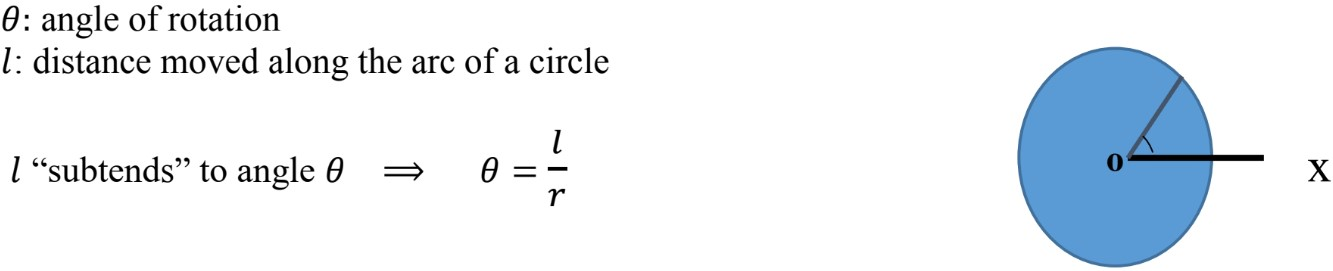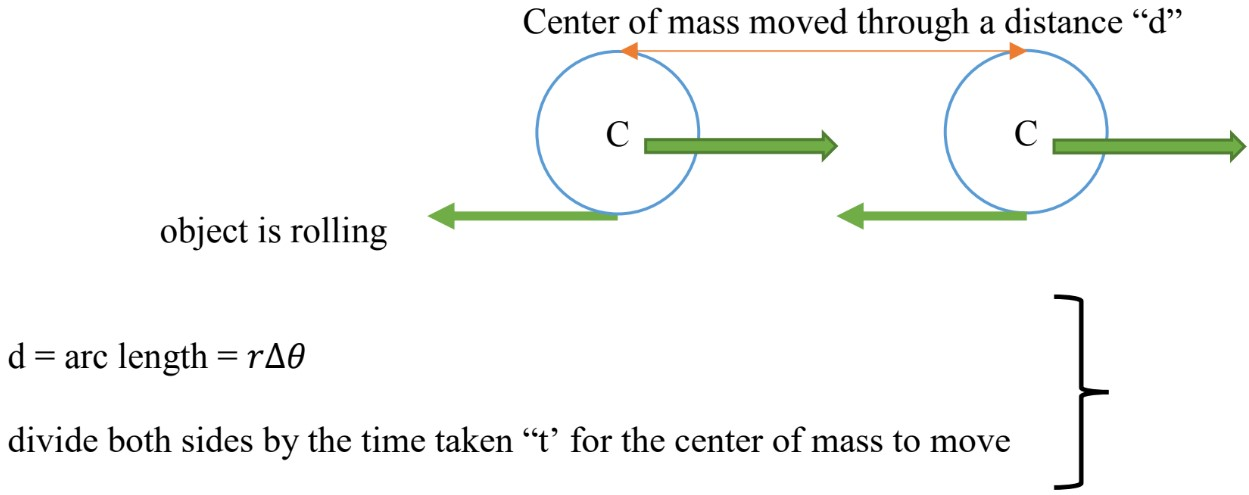# Rotational Vs. translational kinematics#### Everything You Need in One Place

Homework problems? Exam preparation? Trying to grasp a concept or just brushing up the basics? Our extensive help & practice library have got you covered.#### Learn and Practice With Ease

Our proven video lessons ease you through problems quickly, and you get tonnes of friendly practice on questions that trip students up on tests and finals.#### Instant and Unlimited Help

Our personalized learning platform enables you to instantly find the exact walkthrough to your specific type of question. Activate unlimited help now!

##### Intros
###### Lessons
1. Rotational Motion
2. Translational-Rotational Analogues
3. Tangential Acceleration Vs. Centripetal Acceleration
4. Rotational Kinematics Equations
5. Rolling Without Slipping
##### Examples
###### Lessons
1. A centrifuge starts from rest and has an angular acceleration of 150 rad/s2 for 2.5s.
1. Find the rotational displacement and number of revolutions per minute.
2. What is the final angular velocity after 2.5s?
2. A car engine slows down from 4200rpm to 600rpm in 2.0s.
1. Calculate the angular acceleration.
2. Find the total number of revolution the car makes is this time.
3. A high-speed jet takes 1.5 min to turn through 20 complete revolutions before reaching its final speed.
1. What is the angular acceleration?
2. What was the final angular speed in rpm?
4. To move a large pottery wheel with a radius of 22.0cm, a small rubber wheel is used. Wheels are mounted and their circular edges are in contact. The radius of the small wheel is 3.0cm and it accelerates at the rate of 6.4 rad/s2 without slipping.
1. Calculate the angular acceleration of the pottery wheel.
2. How much time does it take for the pottery wheel to reach a speed of 62 rmp?
5. A car reduces its speed uniformly from 86km/h to 44 km/h, during this time the wheels of the car with a diameter of 0.60m, make 66 revolutions.
1. Find the angular acceleration of the wheels.
2. If the car continues to decelerate, how many more revolutions are required to stop the car?
###### Free to Join!
StudyPug is a learning help platform covering math and science from grade 4 all the way to second year university. Our video tutorials, unlimited practice problems, and step-by-step explanations provide you or your child with all the help you need to master concepts. On top of that, it's fun - with achievements, customizable avatars, and awards to keep you motivated.
• #### Easily See Your ProgressWe track the progress you've made on a topic so you know what you've done. From the course view you can easily see what topics have what and the progress you've made on them. Fill the rings to completely master that section or mouse over the icon to see more details.
• #### Make Use of Our Learning Aids###### Practice Accuracy

Get quick access to the topic you're currently learning.

See how well your practice sessions are going over time.

Stay on track with our daily recommendations.

• #### Earn Achievements as You LearnMake the most of your time as you use StudyPug to help you achieve your goals. Earn fun little badges the more you watch, practice, and use our service.
• #### Create and Customize Your AvatarPlay with our fun little avatar builder to create and customize your own avatar on StudyPug. Choose your face, eye colour, hair colour and style, and background. Unlock more options the more you use StudyPug.
###### Topic Notes

In this lesson, we will learn:

• Definition of Rotational Motion, Rigid Object and The Axis of Rotation.
• Translational-Rotational Analogues
• Tangential Acceleration Vs. Centripetal Acceleration
• Rotational Kinematics Equations
• Rolling Without Slipping Motion

Notes:

Rotational Motion
• To explain the rotational motion, we need to consider a rigid object.

• A rigid object: it is an object with a definite shape, the particles composing the object are at a fixed position relative to one another.

• When an object rotates, all the points composing the object move in a circle.

• Axis of Rotation: The center of all circles lie on a line called Axis of Rotation, which is perpendicular to the page.• Through rotation, the object changes position and moves through different angles; $\theta _{i}, \theta _{f}$

• $\Delta \theta = \theta_{f} - \theta_{i}$

$\qquad \Delta \theta$: Change in position, angular displacement (radians)
$\qquad \theta_{f}$: Final position
$\qquad \theta_{i}$: Initial position

• Similar to translational motion velocity is defined as a change in position over time elapsed;

• $\large w = \frac{\Delta \theta}{\Delta t}$

$w$: angular velocity (radians/sec)

• Similarly, the acceleration is defined as a change in velocity over time elapsed;

• $\propto \, =$ $\large \frac{\Delta w}{\Delta t}$

$\propto$: angular acceleration (radians/sec2)

Translational-Rotational Analogues• Each point of a rotating object has a linear velocity and a linear acceleration, so we have to relate the linear quantities to the angular quantitates.$v =$ $\large \frac{\Delta l}{\Delta t}$ $=$ $\large \frac{r \Delta \theta }{\Delta t}$ $= rw$

$v = rw \,$ (1)

$a =$ $\large \frac{\Delta v}{\Delta t}$ $=$ $\large \frac{r \Delta w}{\Delta t}$ $= r \propto$

$a = r \propto \,$ (2)

Tangential Acceleration Vs. Centripetal Acceleration

In rotational motion, we have to consider the two components of acceleration,

$a_{r}$; tangential component, that changes the magnitude of the velocity and it is tangent to the direction of motion;

$a_{r} = r \propto$

$a_{c}$; centripetal competent, that changes the direction of velocity and is directed towards the center (caused by centripetal force);

$a_{c} =$ $\large \frac{v^{2}}{r}$

To find the total acceleration we can find the magnitude of the acceleration using the Pythagoras theorem;

$a^{2}_{total} \, = \, a\frac{2}{T} \, +\, a\frac{2}{c}$

$a_{total} \, = \, \sqrt{a^{2}_{T} \, + \, a^{2}_{c} }$

Rotational Kinematics Equations
The following table compares the rotational kinematics equation to the translational kinematics equation.Conversion Factor
It is very common in rotational motion to measure the angular velocity in round per minutes (rmp), in order to convert to the standard unit of radians per second (rad/s), we use the following the conversion factor;

rmp × $\frac{2 \pi \; rad \; / \; rev}{60\;sec\;sec\; / \; min}$ rad/s

Rolling Without Slipping
There are many examples in everyday life, where the object rolls but it does not slip.
Examples: rolling ball, rolling wheel.
Rolling without slipping depends on the static friction between the rolling object and the floor.
In this case, we have to consider both rotational and translational motion. To relate these two types of motion, use $v = rw$ (where $r$ is the radius).

As the following diagram represents, the wheel is rolling (Rotational Motion) to the right and at the same time, the center of gravity is changing position (Translational Motion).$\large \frac{d}{t}$ $=$ $\large \frac{r \Delta \theta}{t}$ $\quad \Rightarrow \quad v = rw$

$v$: Center of mass speed
$r$: Radius
$w$: Angular speed about the center of mass